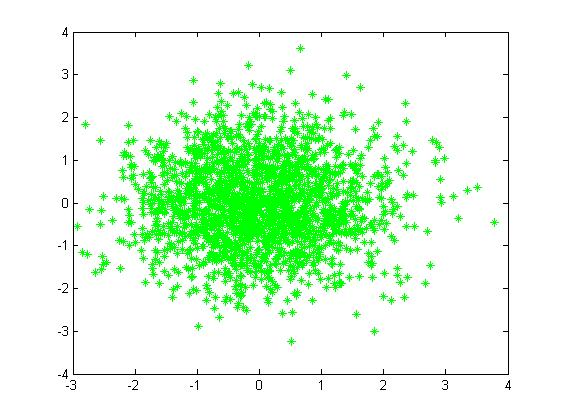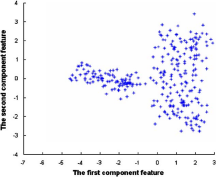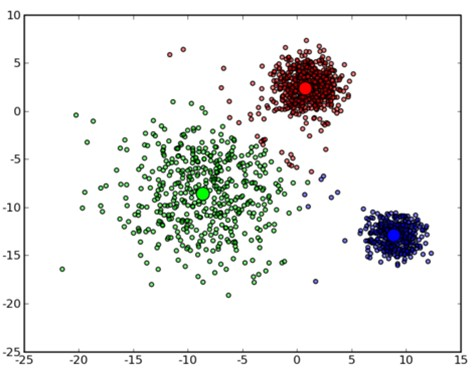机器学习读书笔记（高斯混合模型GMM与EM）（改）

高斯混合模型（Gaussian mixture model，GMM）是单一高斯概率密度函数的延伸。GMM能够平滑地近似任意形状的密度分布。



单高斯分布模型GSM

g(xi;μ,Σ)=1(2π)d|Σ|exp[12(xμ)TΣ1(xμ)]

exp(x1μ,x2μ,....xnμ)协方差矩阵简介（与主体内容无关，仅单纯介绍）

Wikipedia是这么详细定义的：

X=X1Xn

Σij=cov(Xi,Xj)=E[(Xiμi)(Xjμj)]

Σ=E[(XiE|X|)(XjE|X|)]=$\Sigma={\mathrm {E}}{\begin{bmatrix}(X_{i}-E|X|)(X_{j}-E|X|)^{\top }\end{bmatrix}}=$
E[(X1μ1)(X1μ1)]E[(X2μ2)(X1μ1)]E[(Xnμn)(X1μ1)]E[(X1μ1)(X2μ2)]E[(X2μ2)(X2μ2)]E[(Xnμn)(X2μ2)]E[(X1μ1)(Xnμn)]E[(X2μ2)(Xnμn)]E[(Xnμn)(Xnμn)]$\begin{bmatrix}\mathrm {E} [(X_{1}-\mu _{1})(X_{1}-\mu _{1})]&\mathrm {E} [(X_{1}-\mu _{1})(X_{2}-\mu _{2})]&\cdots &\mathrm {E} [(X_{1}-\mu _{1})(X_{n}-\mu _{n})]\\\\\mathrm {E} [(X_{2}-\mu _{2})(X_{1}-\mu _{1})]&\mathrm {E} [(X_{2}-\mu _{2})(X_{2}-\mu _{2})]&\cdots &\mathrm {E} [(X_{2}-\mu _{2})(X_{n}-\mu _{n})]\\\\\vdots &\vdots &\ddots &\vdots \\\\\mathrm {E} [(X_{n}-\mu _{n})(X_{1}-\mu _{1})]&\mathrm {E} [(X_{n}-\mu _{n})(X_{2}-\mu _{2})]&\cdots &\mathrm {E} [(X_{n}-\mu _{n})(X_{n}-\mu _{n})]\end{bmatrix}$

最大似然估计的结果

1. 在单高斯密度函数的假设下，当 x=xi$x=x_i$ 时，其密度密度为 g(xi;μ,Σ)$g(x_{i};\mu,\Sigma)$
2. 若我们假设 xi$x _i$ ,i=1 ~ n 之间为互相独立的事件，则发生 X=x1,x2,...,xn$X={x_1,x_2,...,x_n}$ 的概率密度为:

p(X;μ,Σ)=i=1ng(xi;μ,Σ)

3. 然后就是取对数求导，经计算获得参数μ与Σ的估计
μ^=1ni=1nxiΣ^=1n1i=1n(xiμ^)(xiμ^)T

高斯混合模型GMM

p(x)=α1g(x1;μ1,Σ1)+α2g(x2;μ2,Σ2)+α3g(x3;μ3,Σ3)$p(x)=\alpha_1 g(x_{1};\mu_1,\Sigma_1)+\alpha_2 g(x_{2};\mu_2,\Sigma_2)+\alpha_3 g(x_{3};\mu_3,\Sigma_3)$

(α1,α2,α3,μ1,μ2,μ3,Σ1,Σ2,Σ3)$(\alpha_1,\alpha_2,\alpha_3,\mu_1,\mu_2,\mu_3,\Sigma_1,\Sigma_2,\Sigma_3)$，而且要满足α1+α2+α3=1$\alpha_1+\alpha_2+\alpha_3=1$
α是各模型的系数，这其实是一个加权单位化的思想。下面是n=2的混合高斯模型，我们发现有两个中心点。由于模型增多造成的模型参数增多，确定参数成为首要问题。（看这个图，强迫症的人肯定想聚类一下）Σi=σ2iI,i=1,2,3,...$\Sigma_i=\sigma_i^2I,i=1,2,3,...$

EM算法在高斯混合模型的应用

下面主要摘自李航《统计学习方法》，以及自己的笔记。周志华的《机器学习》书中没有详细介绍。


while（）\\不接受误差就循环
{
Expectation();
Maximization();
}

P(x|θ)=k=1Kαkϕ(x|θk)θ=(α1,α2,...,αK;μ1,μ2,...,μK,σ1,σ2,...,σK)

1. 明确隐变量，写出完全数据的对数似然函数
我们现在先切换到上帝视角。可以设想观测数据xii=1,2,...,N$x_i，i=1,2,...,N$是这样产生的：首先依据各单一模型的系数αk$\alpha_k$选择高斯分布分模型ϕ(x|θk)$\phi(x|\theta_{k})$ ; 然后依据这个分模型的概率分布生成观测数据xi$x_i$。从上图n=2的角度讲就是，选个圈，生成点。
我们从上帝视角回来，事实是我们手上只有不知从哪里冒出来的X数据集，它是已知的，但反应X中的某xi$x_i$是来自哪个分模型是未知的。这TM就很尴尬了。
像这种有结论没原因的事例。我们最简单的方法就是设置一个隐变量。这一步就体现了数学家的高明。这里习惯用隐变量 γ来表征，其定义如下：
γik={10ik

其中i=1,2,…,N;k=1,2,…,K， γik$\gamma_{ik}$就是分模型k对观测数据xi$x_i$的响应度 。
这样写出完全数据的似然函数（后验概率的感觉出现了）：
P(y,γ|θ)=j=1NP(xi,γi1,γi2,...,γik|θ)=k=1Kαnkki=1N[12πσkexp((yiμk)22σ2k)]γik

其中
nk=i=1Nγik
2. EM算法中的E步，确定Q函数，求它的期望

Q函数：完全数据的对数似然函数logP(Y,Z|θ)$logP(Y,Z|θ)$关于在给定观测数据Y和当前参数θ(i)$\theta^{(i)}$下对未观测数据Z的条件概率分布P(Z|Y,θ(i))$P(Z|Y,\theta^{(i)})$的期望称为Q函数。即：

Q(θ,θ(i))=EZ[logP(Y,Z|θ)|Y,θ(i)]

这样的Q函数可以作递推，上面公式中，Z是未观测数据，Y是观测数据，Q函数中第一个变元代表极大化参数，第二个表示参数当前估计值。



Q(θ,θ(i))=E[logP(x,γ|θ)|x,θ(i)]=k=1K{j=1N(Eγjk)logαk+j=1N(Eγjk)[log(12π)logσk12σ2k(yjμk)2]}

γjk^=E(γjk|x,θ)=P(γjk=1|x,θ)=αkϕ(yj|θk)Kk=1αkϕ(yj|θk),j=1,2,...,N;k=1,2,...,K

3. EM中的M步

θ(i+1)=argmaxθQ(θ,θ(i))

k=1Kαk=1
(就是开始时我们说的加权值要等于1)
μk^=Nj=1γ^jkxjNj=1γ^jkσ2k^=Nj=1γ^jk(xjμk)2Nj=1γ^jkαk^=nkN=Nj=1γjk^N

个人总结K-means的第一步开始是明确要聚几类（即K等于几），GMM第一步开始的明确是混合几个高斯模型（即N等于几）。